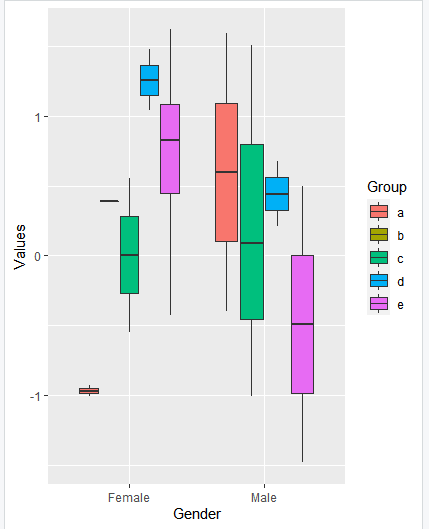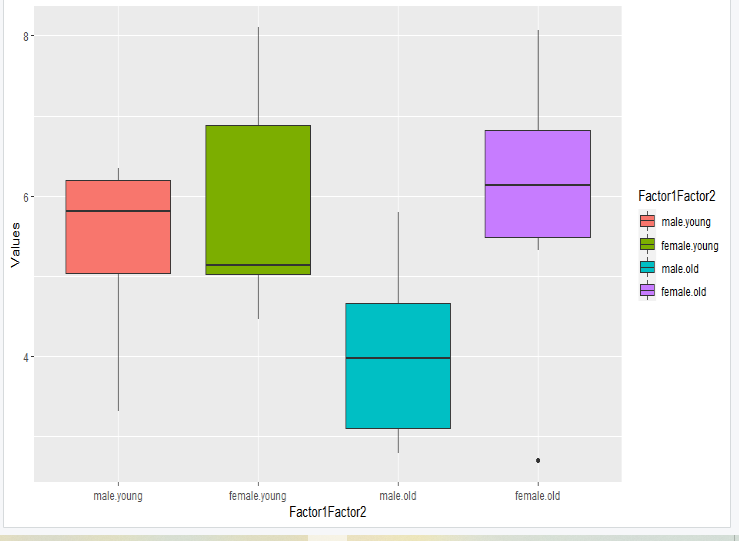Open in App
Not now

# Create Boxplot with respect to two factors using ggplot2 in R

• Last Updated : 18 Jul, 2021

Multiple variable distributions can be visualized with boxplots. ggplot2 allows us to create beautiful boxplots quickly. It is possible to have multiple subgroups for a variable of interest. In those situations, it is very useful to visualize using “grouped boxplots”. The ggplot2 package in R programming language provides a number of options for visualizing such grouped boxplots.

Now talking about Boxplot, then each boxplot is created for every category or level in that factor that is represented by a factor and a numerical column. geom_boxplot also allows us to plot both factors using the fill argument when there are two factors. Geom_boxplot() is the key function

Syntax

geom_boxplot(width,notch,color,size,linetype, fill,outliner.color, outliner.size, outliner.shape)

Parameter:

• width: width of the boxplot
• notch: if it is true then it will create a notched boxplot and notches are used to compare boxplots.
• color, size,line type: borderline, color, size and shape.
• fill: used to fill box plot areas.
• outlier.colour, outlier.shape, outlier.size: The color, the shape and the size for outlying points.

Now let us look at few implementations.

Example 1:

## R

 `# create a Data Frame``Gender<-``sample``(``c``(``"Male"``,``"Female"``),20,replace=``TRUE``)``Values<-``rnorm``(20,mean=0,sd=1)``Group<-``sample``(``letters``[1:5],20,replace=``TRUE``)`` ` `df<-``data.frame``(Gender,Values,Group)`` ` `library``(ggplot2)`` ` `# creating a boxplot``ggplot``(df,``aes``(Gender,Values))+``geom_boxplot``(``aes``(fill=Group))`

Output:Example 2:

## R

 `# load ggplot2 package if already installed``library``(ggplot2)`` ` `# create a data frame with two factors ``df <- ``data.frame``(Factor1=``factor``(``rbinom``(30, 1, 0.55), ``                                ``label=``c``(``"male"``,``"female"``)), ``                   ``Factor2=``factor``(``rbinom``(30, 1, 0.45), ``                                  ``label=``c``(``"young"``,``"old"``)),``                   ``Values=``rnorm``(30,mean=5,sd=2))`` ` `# Now make a interaction between two factors ``# on x axis  ``df\$Factor1Factor2 <- ``interaction``(df\$Factor1, df\$Factor2) `` ` `# now Plot Boxplot with fill color according``# to factor1 and factor2``ggplot``(``aes``(y = Values, x = Factor1Factor2), data = df) + ``geom_boxplot``(``aes``(fill=Factor1Factor2))``  `

Output:My Personal Notes arrow_drop_up